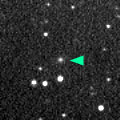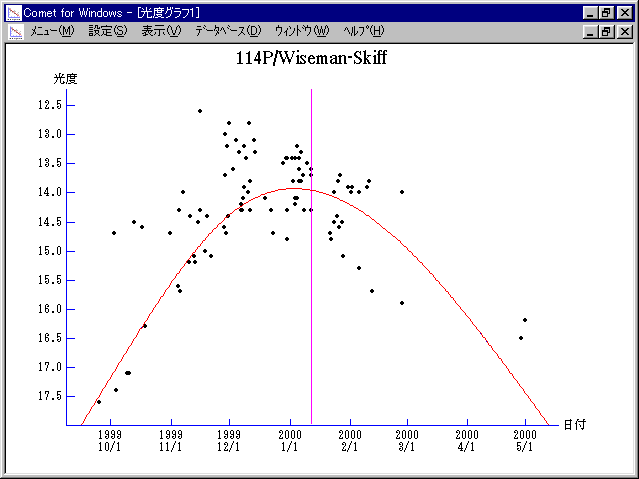# \$B%o%\$%9%^%s(B-\$B%9%-%C%UWB@1(B

114P/Wiseman-Skiff (2000)

 English version Home page Updated on September 23, 2006###\$B###\$B50F;MWAG(B

```   The following improved orbital elements by Kenji Muraoka,
are from 238 observations 1986 to 2000, including 9 Planets,
Moon and 5 minor planets perturbations and non-gravitational
effect of style II.  The mean residual is +/- 0.69 arc seconds.

Epoch  =  2000 Jan  = 2451560.5
T  =  2000 Jan. 11.73069       +/- 0.00047 (m.e.) TT
Peri. =  172.81818                +/- 0.00031
Node  =  271.06994                +/- 0.00003   (2000.0)
Incl. =   18.29087                +/- 0.00002
q  =    1.5694441              +/- 0.0000012 AU
e  =    0.5564860              +/- 0.0000007
a  =    3.5386578              +/- 0.0000018 AU
n  =    0.14806289             +/- 0.00000011
P  =    6.657                  +/- 0.0000050  years
A1  =   +0.641                  +/- 0.041
A2  =   +0.00944                +/- 0.00116
```

###\$B@1?^(B###\$B8wEYJQ2=(B

```        m1 = 9.0 + 5 log\$B&\$(B + 27.0 log r(t - 20)
```##### \$B50F;MWAG\$OB<2,7r<#;a\$N7W;;\$K\$h\$k\$b\$N\$G\$9!#(B \$B@1?^\$O(B StellaNavigator Ver.2.0 for Windows (\$B%"%9%H%m%"!<%D(B \$BJTCx(B / \$B%"%9%-!<=PHG6I4)(B) \$B\$G:n@.\$7\$?\$b\$N\$G\$9!#(B \$B8wEY%0%i%U\$O(BComet for Windows\$B\$G:n@.\$7\$?\$b\$N\$G\$9!#(B